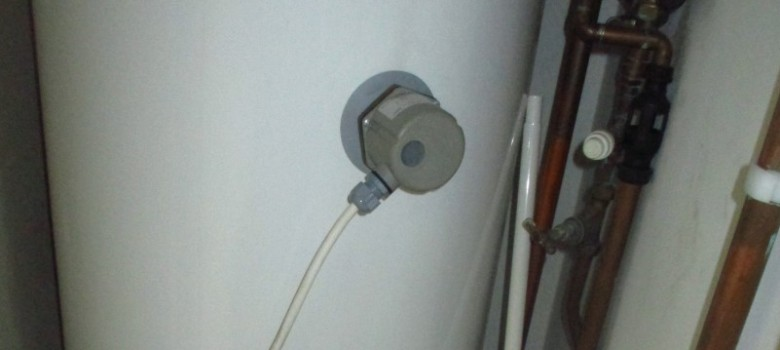If you have ever looked at your electricity bill you will see that electricity is measured in kiloWatt hours (or kWh). A kWh is a unit of energy equivalent to one kW of power expended for one hour.

An average 3 bed home will use approximately 4,000 kWh of electricity per year; that includes things like lighting, watching TV and running a dishwasher.

In some cases, people heat their water with electricity – normally through the use of electric immersion heaters that act like huge like kettles.  We often get asked whether these are expensive to run, so the following should help you work that out for yourselves!

## How many KW of electricity are needed to heat a hot water tank?

So the two bits of information you will need to help calculate this are the size of the tank measured in litres, and the required temperature rise.The required temperature rise (measured in centigrade) is calculated by taking the starting temp of your cold water from the hot water temperature required. For example, if your water is 100C and you want to heat it to 600C, then the temperature rise would be 500C.

The following formula can then be used to calculate the power (in kW) needed to heat a specific volume of water by a given temperature in 1 hour.

Volume of tank x 4 x temperature rise / 3412 = Power required

So if the tank is 125 litres and you want to take the water up to 650C from 100C, then it would simply be:

124 x 4 x 55 / 3412 = 8kW

This means that you would need 8kW of electricity to heat this volume of water in 1 hour. If you wanted to heat it in 30 mins you would need 16kW of power, and so forth.

## Immersion heaters are measured in kW

When you buy an immersion heater it is given a kW rating, for example 3kW or 6kW.

If you take the example of required temperature rise above and you have a 3kW immersion heater it would take about 2hrs 30 to heat the water (3kW each hour, so in two and a half hours that would give 7.5kW).

## Cost of running an immersion heater

The cost of electricity is about 14p/kWh, which means that it would cost about 45p to run a 3kW immersion heater for an hour.

Normally though, the immersion will turn on and off via the thermostat so the amount of time they are on fluctuates, depending on the existing temperature of the water in the hot water tank.

Hopefully the above has been useful and you can apply it to your home! Please remember that hot water should be heated to at least 600C in order to kill legionella bacteria!

Have a question or would like to find out more?